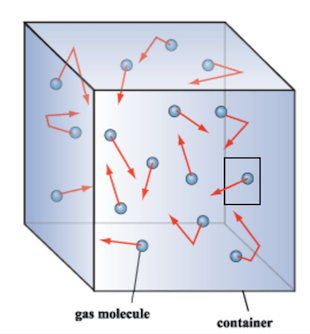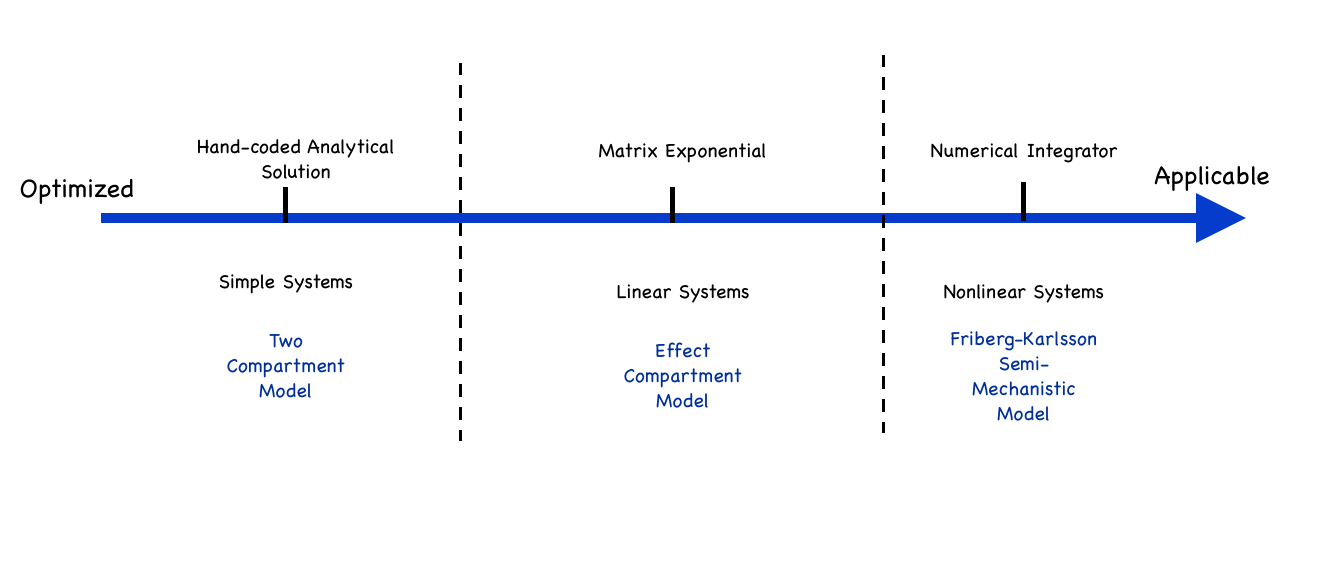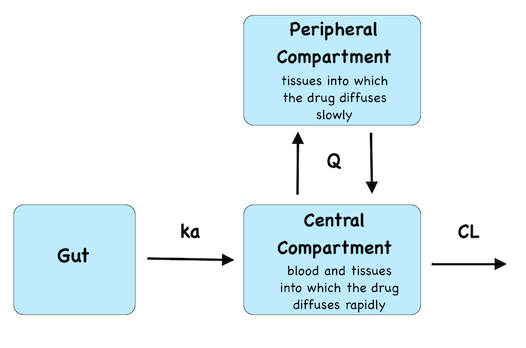## 1. Introduction

Differential Equations can help us model sophisticated processes in biology, physics, and many other fields. Over the past year, the Stan team has developed many tools to tackle models based on differential equations.

### 1.1 Why Use Ordinary Differential Equations (ODEs)?

We deal with an ODE when we want to determine a function $$y(t)$$ at a specific time but only know the derivative of that function, $$\frac{dy}{dt}$$. In other words, we know the rate at which a quantity of interest changes but not the quantity itself. In many scenarios, the rate depends on the quantity itself.

To get a basic intuition, let us consider an example. Imagine a gas container with a hole in it. We can think of the gas as being made of molecules that move randomly in the container. Each molecule has a small chance of leaking through the hole. Thus the more molecules there are inside the container, the higher the number of escaping molecules per unit time.If there are a large number of molecules, and the gas behaves like a continuous fluid, we’ll observe that the more gas in the container, the higher the leakage. This statement can be written as the differential equation:

$\frac{dy}{dt} = -ky(t)$

where $$k$$ is a positive constant.

In this presentation, I’ll use examples from pharmacometrics to motivate the use of differential equations. Pharmacometrics is the quantitative study of how drug compounds circulate in and affect the patient’s body.

In a compartment model, we treat physiological components, such as organs, tissues, and circulating blood, as compartments between which the drug flows and/or in which the drug has an effect.

A compartment may refer to more than one physiological component. For example, the central compartment typically consists of the systemic circulation (the blood) plus tissues and organs into which the drug diffuses rapidly.

Just like our leaking gas in a container, the rate at which the drug amount changes depends on the drug amount in the various compartments. Things are slightly more complicated because instead of one box, we now deal with a network of containers. This results in a system of differential equations, where each equation describes the drug amount in one compartment.

### 1.2 Tools for Solving ODEs

Solving ODEs can be notoriously hard.

In the best case scenario, an ODE system has an analytical solution we can hand-code. That is, we figure $$y(t)$$ on paper and write the solution in the Stan file. You should always do this when possible, because it saves the computer the expensive task of solving ODEs.

The vast majority of times, we need to approximate the solution numerically. There exists a very nice technique, involving matrix exponentials, for solving linear ODEs. Nonlinear systems are significantly more difficult but fortunately we can tackle these problems with numerical integrators.

Specialized algorithms for solving ODEs tend be more efficient but have a narrower application; the reverse holds for more general tools. Stan provides both, thereby allowing users to tackle a broad range of problems and optimize their model when possible.

### 1.3 Overview of Cases

We present three models, based on three different types of ODE systems:

1. A system with an analytical solution we code by hand
2. A linear system we solve using a matrix exponential solution
3. A nonlinear system we integrate numerically

For each model, we will use a different technique to solve differential equations.Note the ODE system only describes the natural evolution of the patient’s system, i.e, how does the drug behave once it is already in the body. It does not account for outside interventions, such as drug intake. To be accurate, our models must compute these exterior events and solve ODEs in the context of an event schedule.

All our examples are fitted to simulated data, generated with the R package mrgsolve .

## 2. ODE System with an Analytical Solution

Consider the common scenario in which a patient orally takes a drug. The drug enters the body through the gut and is then absorbed into the blood. From there it diffuses into and circulates back and forth between various tissues and organs. Over time, the body clears the drug, i.e. the drug exits the body (for instance through urine).

Our model divides the patient’s body into three compartments:

1. The absorption compartment: the gut
2. The central compartment: the systemic circulation (blood) and tissues/organs into which the drug diffuses rapidly
3. The peripheral compartment: other tissues/organs into which the drug distributes more slowly

We conventionally call this a Two Compartment Model, which is unfortunate because the model has three compartments. The idea is that the “absorption compartment” doesn’t really count. We’ll adopt this convention to agree with the community.We describe the drug absorption with the following differential equations:

$\frac{dy_{\mathrm{gut}}}{dt} = -k_a y_{\mathrm{gut}} \\ \frac{dy_{\mathrm{central}}}{dt} = k_a y_{\mathrm{gut}} - (\frac{CL}{V_{\mathrm{central}}} + \frac{Q}{V_{\mathrm{central}}}) y_{\mathrm{central}} + \frac{Q}{V_{\mathrm{peripheral}}} y_{\mathrm{peripheral}} \\ \frac{dy_{\mathrm{peripheral}}}{dt} = \frac{Q}{V_{\mathrm{central}}} y_{\mathrm{central}} - \frac{Q}{V_{\mathrm{peripheral}}} y_{\mathrm{peripheral}}$

whith
$$y_{\mathrm{gut}}$$ : the drug amount in the gut (mg)
$$y_{\mathrm{central}}$$ : the drug amount in the central compartment (mg)
$$y_{\mathrm{peripheral}}$$ : the drug amount in the peripheral compartment (mg)
$$k_a$$ : the rate constant at which the drug flows from the gut to the central compartment ($$h^{-1}$$)
$$Q$$ : the clearance at which the drug flows back and forth between the central and the peripheral compartment (L/h)
$$CL$$ : the clearance at which the drug is cleared from the central compartment (L/h)
$$V_{\mathrm{central}}$$ : the volume of the central compartment (L)
$$V_{\mathrm{peripheral}}$$ : the volume of the peripheral compartment (L)

The data we fit our model to is the drug concentration in the blood, which our model treats as the concentration in the central compartment, and is given by:

$c = \frac{y_{\mathrm{central}}}{V_{\mathrm{central}}}$

and the parameters we wish to estimate are $$k_a$$, $$Q$$, $$CL$$, $$V_{\mathrm{central}}$$, and $$V_{\mathrm{peripheral}}$$.

### 2.1 Stan Code

We’ll first write a function that returns the solution to the ODEs at a time $$t_0 + dt$$. This function requires us to specify the initial state of the system at $$t_0$$. Since we know the analytical solution, we’ll hand-code it (even though this requires a fair amount of work).

Remember the ODEs describe a physiological process, but they do not tell us anything about the treatment the patient receives. We typically think of a clinical trial as a series of events, such as a drug intake or a blood sampling for observation. Together these events make up the event schedule.

Some of the arguments in the following function, notably $$amt$$, $$cmt$$, and $$evid$$, are terms in pharmacometrics used to characterize the clinical treatment a patient recieves. Defining them is beyond the scope of this notebook, but it is worth pointing out these arguments come into play only when an exterior intervention perturbs the natural evolution of the system.

  vector twoCptModel1(real dt, vector init, real amt, int cmt, int evid,
real CL, real Q, real V1, real V2, real ka){
vector x;
real k10;
real k12;
real k21;
real ksum;
vector a;
vector alpha;

k10 = CL / V1;
k12 = Q / V1;
k21 = Q / V2;
ksum = k10 + k12 + k21;
alpha = (ksum + sqrt(ksum * ksum - 4.0 * k10 * k21))/2.0;
alpha = (ksum - sqrt(ksum * ksum - 4.0 * k10 * k21))/2.0;
alpha = ka;

x = rep_vector(0.0, 3);

if(init != 0.0){
x = init * exp(-alpha * dt);
a = ka * (k21 - alpha) / ((ka - alpha) * (alpha - alpha));
a = ka * (k21 - alpha) / ((ka - alpha) * (alpha - alpha));
a = -(a + a);
x = init * sum(a .* exp(-alpha * dt));
a = ka * k12 / ((ka - alpha) * (alpha - alpha));
a = ka * k12 / ((ka - alpha) * (alpha - alpha));
a = -(a + a);
x = init * sum(a .* exp(-alpha * dt));
}

if(init != 0){
a = (k21 - alpha) / (alpha - alpha);
a = (k21 - alpha) / (alpha - alpha);
x = x + init * sum(segment(a, 1, 2) .* exp(-segment(alpha, 1, 2) * dt));
a = k12 / (alpha - alpha);
a = -a;
x = x + init * sum(segment(a, 1, 2) .* exp(-segment(alpha, 1, 2) * dt));
}

if(init != 0){
a = k21 / (alpha - alpha);
a = -a;
x = x + init * sum(segment(a, 1, 2) .* exp(-segment(alpha, 1, 2) * dt));
a = (k10 + k12 - alpha) / (alpha - alpha);
a = (k10 + k12 - alpha) / (alpha - alpha);
x = x + init * sum(segment(a, 1, 2) .* exp(-segment(alpha, 1, 2) * dt));
}

if(evid == 1) x[cmt] = x[cmt] + amt;

return x;
}

In this next piece of code, we write a function that handles the event schedule and calls the function we just defined:

  matrix twoCptModel(real[] time, real[] amt, int[] cmt, int[] evid,
real CL, real Q, real V1, real V2, real ka){
vector init;
real dt;
real t0;
matrix[size(time), 3] result;
int nt;

nt = size(time);

init = rep_vector(0, 3);
t0 = time;
for(i in 1:nt){
dt = time[i] - t0;
init = twoCptModel1(dt, init, amt[i], cmt[i], evid[i],
CL, Q, V1, V2, ka);
for(j in 1:3) result[i, j] = init[j];
t0 = time[i];
}
return result;
}

The full model file can be found under models/twoCpt/twoCpt.stan.

### 2.2 R Script

We fit the model to the data using 4 MCMC chains, each with a different initial parameter estimate. We compute 1000 warm-up iterations per chain to explore the parameter space, followed by another 1000 iterations to sample from the approximated posterior distribution.

Read in data and create initial estimates:

data <- read_rdump(file.path(modelDir, paste0(modelName,".data.R")))

## initial estimates will be generated randomly for each chain
init <- function(){
list(CL = exp(rnorm(1, log(10), 0.2)),
Q = exp(rnorm(1, log(20), 0.2)),
V1 = exp(rnorm(1, log(70), 0.2)),
V2 = exp(rnorm(1, log(70), 0.2)),
ka = exp(rnorm(1, log(2), 0.2)),
ke0 = exp(rnorm(1,log(1),0.2)),
EC50 = exp(rnorm(1,log(100),0.2)),
sigma = 0.5,
sigmaResp = 20)
}

Fit the model:

## Specify the variables for which you want history plots
parametersToPlot <- c("CL", "Q", "V1", "V2", "ka", "sigma")

otherRVs <- c("cObsPred")

parameters <- c(parametersToPlot, otherRVs)
parametersToPlot <- c("lp__", parametersToPlot)

nChains <- 4
nPost <- 1000 ## Number of post-warm-up samples per chain after thinning
nBurn <- 1000 ## Number of warm-up samples per chain after thinning
nThin <- 1
nIter <- (nBurn + nPost) * nThin
nBurnin <- nBurn * nThin

fit <- stan(file = file.path(modelDir, paste(modelName, ".stan", sep = "")),
data = data,
pars = parameters,
iter = nIter,
warmup = nBurnin,
thin = nThin,
init = init,
chains = nChains,
cores = min(nChains, parallel::detectCores()))
## In file included from /data/StanConMargossian/lib/BH/include/boost/config.hpp:39:0,
##                  from /data/StanConMargossian/lib/BH/include/boost/math/tools/config.hpp:13,
##                  from fileeef4bbca430.cpp:8:
## /data/StanConMargossian/lib/BH/include/boost/config/compiler/gcc.hpp:186:0: warning: "BOOST_NO_CXX11_RVALUE_REFERENCES" redefined [enabled by default]
##  #  define BOOST_NO_CXX11_RVALUE_REFERENCES
##  ^
## <command-line>:0:0: note: this is the location of the previous definition
save(fit, file = file.path(modelDir, paste(modelName, "Fit.Rsave", sep = "")))

### 2.3 Diagnostics

Let’s test whether the chains converged to a common distribution for all the key parameters. We include lp__ in our analysis, the log posterior. lp__ varies quickly and is susceptible to outliers, which makes it an interesting parameter to monitor when testing for convergence.

stan_trace(fit, parametersToPlot)